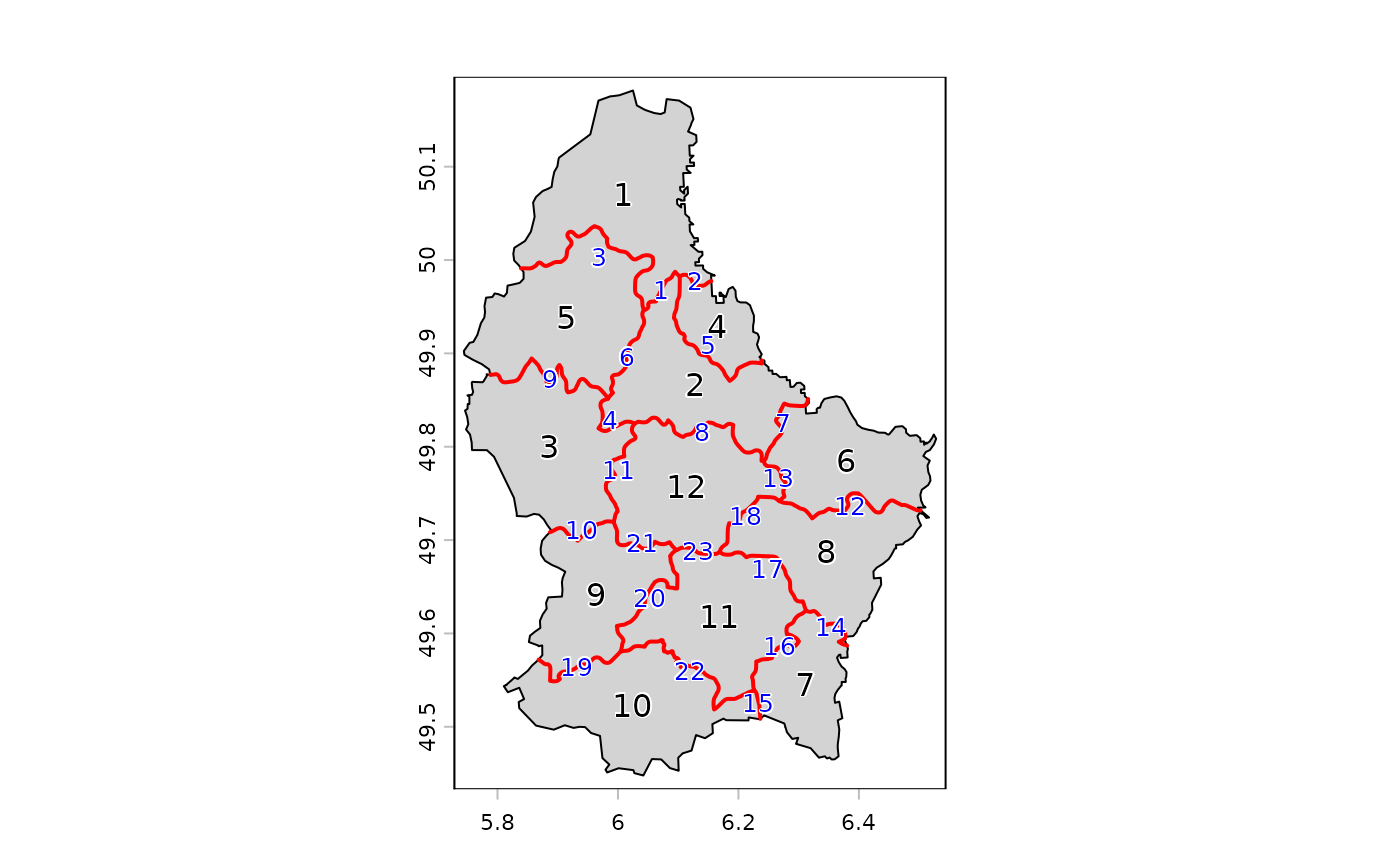Get shared paths of line or polygon geometries. This can for geometries in a single SpatVector, or between two SpatVectors

## Usage

# S4 method for SpatVector
sharedPaths(x, y=NULL)

## Arguments

x

SpatVector of lines or polygons

y

missing or SpatVector of lines or polygons

## Value

SpatVector

gaps, topology

## Examples

f <- system.file("ex/lux.shp", package="terra")
v <- vect(f)
plot(v, col="light gray")
text(v, halo=TRUE)

x <- sharedPaths(v)
lines(x, col="red", lwd=2)
text(x, col="blue", halo=TRUE, cex=0.8)